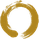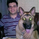5582 vizualizações
5582
Script which calculates the linear regression slope, giving an idea where enter or exit in a market position.
Please if anybody have suggestions, let me know.
```// Created by emiliolb -- Version 1.0
// Contact emiliolb@outlook.com
study(title="Linear Regression Slope", shorttitle="LRS", overlay=true)

src = close
len = input(defval=150, minval=1, title="Linear Regression Length")
lrc = linreg(src, len, 0)
plot(lrc, color = red, title = "Linear Regression Curve", style = line, linewidth = 2)

lrprev = linreg(close, len, 0)
slope = ((lrc - lrprev) / interval)

//Please if somebody have suggestions how show this better, let me know
codiff = slope
plotarrow(codiff, colorup=teal, colordown=orange, transp=40)```Hi,

I'm trying to code a Linear Regression Band indicator that has the same standard deviations as the built in Linear Regression indicator/drawing tool.

The mean of my indicator (code below) is correct but the standard deviation bands are always in the wrong place when I compare them to the same length LR indicator or drawing tool.

Does anyone know how to fix this issue so that the bands match the built in drawing tool/indicator?

Here is my code ...

//@version=3
study(shorttitle="LRB", title="Linear Regression Bands", overlay=true)
source = close
length = input(50, minval=1)
offset = 0
mult = input(2, title="StDev", minval=0.001, maxval=5)
basis = linreg(source, length, offset)
dev = mult * stdev(source, length)
upper = basis + dev
lower = basis - dev
plot(basis, color=red)
plot(upper, color=blue)
plot(lower, color=blue)

Many thanks! Pete
Respostacoondawg71
Hi! I am sorry by delay, I saw now your message. I think the arrow length depends on the spread between high and low candle values, not 100% by very close
I am glad you like the tool, I am newbie in this on trading, I am learning on the fly these things.
Thanks!
Emilio
Respostaemiliolb
I looked up the code and this sentence determine the arrow's length "plotarrow(slope, colorup=teal, colordown=orange, transp=40)"
Resposta